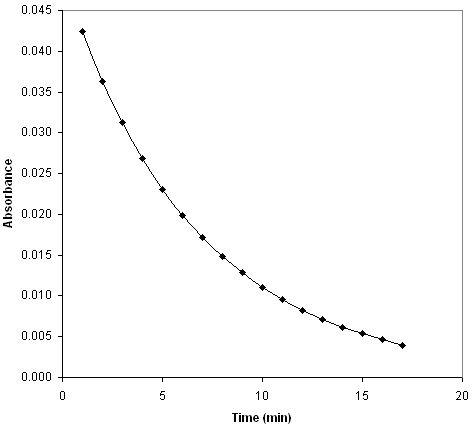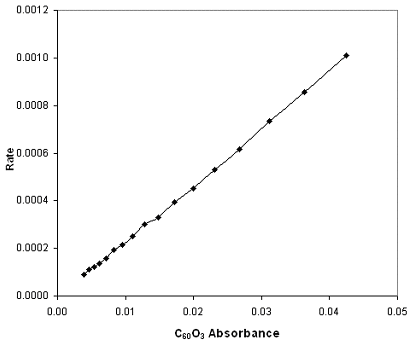## Experimental Determination of Reaction Rates

#### Learning Objective

• Design experiments that use either chemical or physical properties to measure reaction rates

#### Key Points

• Physical measurements can be performed on a system while it is reacting. These measurements have the advantage of not disturbing the system, and they can be taken during the reaction. Examples of these measurements are changes in volume, temperature, and absorption of a solution.
• Chemical methods of measurement yield concentrations directly. A small sample is extracted from the reacting mixture, and the reaction is halted by dilution, cooling the mixture long enough to measure concentrations, or adding another reagent to stop the reaction.
• For zero-order reactions, graph concentration vs. time to get a line with the slope -k. For first-order reactions, graph the logarithm of concentration vs. time to get a line with the slope -k. For second-order reactions, graph the reciprocal of concentration vs. time to get a line of slope -k.

#### Terms

• Beer’s lawRelates the attenuation of light to the properties of the material through which the light is traveling.
• absorbanceA logarithmic measure of the amount of light that is absorbed when passing through a substance.

## Experimental Methods for Measuring Reactions

In order to experimentally determine reaction rates, we need to measure the concentrations of reactants and/or products over the course of a chemical reaction. If we know the order of the reaction, we can plot the data and apply our integrated rate laws. For example, if the reaction is first-order, a plot of ln[A] versus t will yield a straight line with a slope of –k.

There are two main ways to measure the concentrations of reactions: by measuring the changes in an observable physical property, or by taking samples of the reaction solution and measuring concentration directly.

## Physical Measurements

Physical measurements can be performed on the system while it is reacting. These measurements have the advantage of not disturbing the reacting system, and they can usually be measured quickly. For example, if the total number of moles of gas changes during a gas reaction, the course of the reaction can be measured by monitoring the change in pressure at a constant volume. Other physical measurements include optical methods, such as measuring the change in light polarization, the change in the refractive index of the solution, or quite commonly, the change in the color of the solution, and therefore the absorption spectrum. Common electrical methods include changes in the conductivity of a solution, the electrical potential in a cell, and mass spectrometry. Other methods include thermal conductivity, viscosity, heats of reaction, and freezing points.

## Chemical Measurements

Chemical methods yield concentrations directly. A small sample is extracted from the reacting mixture, and the reaction is halted either by dilution, by cooling the mixture, or by adding another chemical reagent to stop the reaction. One disadvantage of this method is that removing a part of the reacting system, or adding an additional reagent to it, gradually changes it over time. Also, there is a time lag between when the sample is taken and when the reaction is measured, which makes the measurement less accurate.

## Using Beer’s Law to Measure Concentration Over Time

One oxidized form of buckminsterfullerene (C60) is C60O3. When a solution of C60O3 is warmed, the compounded decomposes, releasing O2 and creating C60O. The reaction is given by:

$C_{60}O_3\rightarrow O_2+C_{60}O$

The rate of this reaction can be monitored by measuring the absorbance of the solution. The absorbance is given by Beer’s law:

$A=abC$

In this equation, a is the absorptivity of a given molecules in solution, which is a constant that is dependent upon the physical properties of the molecule in question, b is the path length that travels through the solution, and C is the concentration of the solution. We’re interested in determining C.

By running the reaction of interest inside a spectrometer, the absorbance of the solution can be measured over time. The data can then be plotted.Oxidized buckminsterfullerene absorbanceThe absorbance is proportional to the concentration of the C60O3 in solution, so observing the absorbance as a function of time is essentially the same as observing the concentration as a function of time.

By Beer’s law, the absorbance of the solution is directly proportional to the concentration of the C60O3 in solution, so observing the absorbance as a function of time is essentially the same as observing the concentration as a function of time. In this case, the rate law is given by:

$rate=k[C_{60}O_3]$

Therefore, a plot of the rate versus the absorbance will yield a straight line with a slope of k.Reaction rate vs. absorbanceThe absorbance is directly proportional to the concentration, so this is simply a plot of the rate law, rate = k[C60O3], and the slope of the line is the rate constant, k.

## Plotting Integrated Rate Laws to Determine Reaction Rate

As discussed in a previous concept, plots derived from the integrated rate laws for various reaction orders can be used to determine the rate constant k. Recall that for zero-order reactions, a graph of [A] versus time will be a straight line with slope equal to –k. For first-order reactions, a graph of ln[A] versus time yields a straight line with a slope of –k, while for a second-order reaction, a plot of 1/[A] versus t yields a straight line with a slope of k.